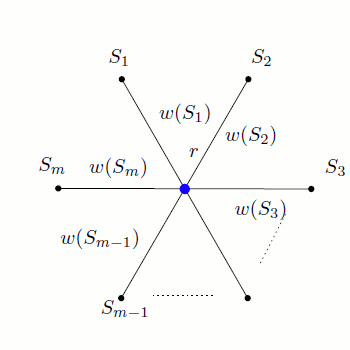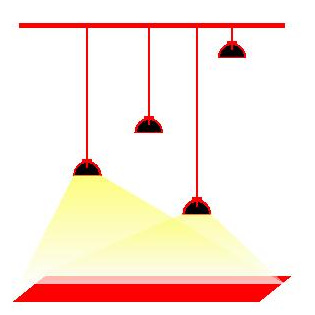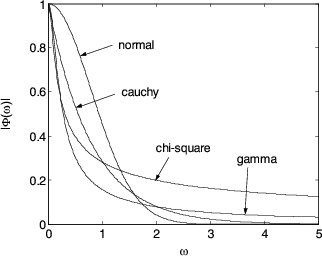## Journal Publications

Khaled Elabssioni, Slobodan Jelić, Domagoj Matijević
The relation of Connected Set Cover and Group Steiner Tree
Theoretical Computer Science, Volume 438, June 2012, Pages 96–101, PDF
 ABSTRACT: We report that the Connected Set Cover (CSC) problem is just a special case of the Group Steiner Tree (GST) problem. Based on that we obtain the first algorithm for CSC with polylogarithmic approximation guarantee as well as the first approximation algorithms for the weighted version of the problem and the version with requirements. Moreover, we argue that the inapproximability result of GST will carry on to the weighted version of the CSC problem.## Other Publications

Slobodan Jelić, Domagoj Matijević
Stage Illumination Problem
published as part of Wolfram Demonstrations Project, 2009.
 ABSTRACT: Consider the following illumination problem: given a stage represented by a horizontal line segment and a set of light sources represented by a set of points in the plane above, assign powers to the light sources such that every point on the stage receives a sufficient amount (say one unit) of light while minimizing the overall power consumption. Under the assumption that the amount of light arriving from a fixed light source decreases rapidly with the distance from the light source, this becomes an interesting optimization problem. Two approximation algorithms based on linear programming are used in this Demonstration.Zvonimir Ivančević, Slobodan Jelić
Application of SVD in information retrieval and image compression (in Croatian)
to appear in Osijek Mathematical Gazette
 ABSTRACT: In this paper the application of singular value decomposition (SVD) in low rank approx- imation of matrix is given. Image compression is performed by taking only first k singular values in SVD of matrix. Illustration of information retrieval is based on the concept of latent semantic indexing model (LSI). Database is determined by matrix whose columns represent documents. Database matrix is substituted with low rank approximation matrix, while searching for relevant documents with respect to user's query is based on calculating the cosine of angle between query and document vectors. Lesser is the angle, a document is more relevant for given query.Slobodan Jelić
Application of Characteristic Functions in Statistics (in Croatian)
to appear in Osijek Mathematical Gazette 10:1, Osijek, 2010.
 ABSTRACT: In this paper cumulative distribution functions of arithmetic mean of random sam- ple from standard normal and standard uniform distributions are calculated by using characteristic functions. An application of basic theoretical results of characteristic functions is illustrated in example of geometric mean of random sample from standard uniform distribution. Some special examples of calculating frequently used statistics are also presented.## Refereed Proceedings

The relation of Connected Set Cover and Group Steiner Tree
Slobodan Jelić, Domagoj Matijević
ApplMath11, 7th Conference on Applied Mathematics and Scientific Computing, 2011 June 13-17, Trogir, Croatia
PDF (slides)